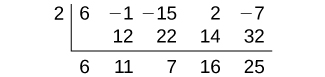# 5.5 Zeros of polynomial functions

 Page 1 / 14
In this section, you will:
• Evaluate a polynomial using the Remainder Theorem.
• Use the Factor Theorem to solve a polynomial equation.
• Use the Rational Zero Theorem to find rational zeros.
• Find zeros of a polynomial function.
• Use the Linear Factorization Theorem to find polynomials with given zeros.
• Use Descartes’ Rule of Signs.
• Solve real-world applications of polynomial equations

A new bakery offers decorated sheet cakes for children’s birthday parties and other special occasions. The bakery wants the volume of a small cake to be 351 cubic inches. The cake is in the shape of a rectangular solid. They want the length of the cake to be four inches longer than the width of the cake and the height of the cake to be one-third of the width. What should the dimensions of the cake pan be?

This problem can be solved by writing a cubic function and solving a cubic equation for the volume of the cake. In this section, we will discuss a variety of tools for writing polynomial functions and solving polynomial equations.

## Evaluating a polynomial using the remainder theorem

In the last section, we learned how to divide polynomials. We can now use polynomial division to evaluate polynomials using the Remainder Theorem    . If the polynomial is divided by $\text{\hspace{0.17em}}x–k,\text{\hspace{0.17em}}$ the remainder may be found quickly by evaluating the polynomial function at $\text{\hspace{0.17em}}k,\text{\hspace{0.17em}}$ that is, $\text{\hspace{0.17em}}f\left(k\right)\text{\hspace{0.17em}}$ Let’s walk through the proof of the theorem.

Recall that the Division Algorithm    states that, given a polynomial dividend $\text{\hspace{0.17em}}f\left(x\right)\text{\hspace{0.17em}}$ and a non-zero polynomial divisor $\text{\hspace{0.17em}}d\left(x\right)\text{\hspace{0.17em}}$ where the degree of $\text{\hspace{0.17em}}\text{\hspace{0.17em}}d\left(x\right)\text{\hspace{0.17em}}$ is less than or equal to the degree of $\text{\hspace{0.17em}}f\left(x\right)$ , there exist unique polynomials $\text{\hspace{0.17em}}q\left(x\right)\text{\hspace{0.17em}}$ and $\text{\hspace{0.17em}}r\left(x\right)\text{\hspace{0.17em}}$ such that

$\text{\hspace{0.17em}}f\left(x\right)=d\left(x\right)q\left(x\right)+r\left(x\right)$

If the divisor, $\text{\hspace{0.17em}}d\left(x\right),\text{\hspace{0.17em}}$ is $\text{\hspace{0.17em}}x-k,\text{\hspace{0.17em}}$ this takes the form

$f\left(x\right)=\left(x-k\right)q\left(x\right)+r$

Since the divisor $\text{\hspace{0.17em}}x-k\text{\hspace{0.17em}}$ is linear, the remainder will be a constant, $\text{\hspace{0.17em}}r.\text{\hspace{0.17em}}$ And, if we evaluate this for $\text{\hspace{0.17em}}x=k,\text{\hspace{0.17em}}$ we have

$\begin{array}{ccc}\hfill f\left(k\right)& =& \left(k-k\right)q\left(k\right)+r\hfill \\ & =& 0\cdot q\left(k\right)+r\hfill \\ & =& r\hfill \end{array}$

In other words, $\text{\hspace{0.17em}}f\left(k\right)\text{\hspace{0.17em}}$ is the remainder obtained by dividing $\text{\hspace{0.17em}}f\left(x\right)\text{\hspace{0.17em}}$ by $\text{\hspace{0.17em}}x-k.\text{\hspace{0.17em}}$

## The remainder theorem

If a polynomial $\text{\hspace{0.17em}}f\left(x\right)\text{\hspace{0.17em}}$ is divided by $\text{\hspace{0.17em}}x-k,\text{\hspace{0.17em}}$ then the remainder is the value $\text{\hspace{0.17em}}f\left(k\right).\text{\hspace{0.17em}}$

Given a polynomial function $\text{\hspace{0.17em}}f,$ evaluate $\text{\hspace{0.17em}}f\left(x\right)\text{\hspace{0.17em}}$ at $\text{\hspace{0.17em}}x=k\text{\hspace{0.17em}}$ using the Remainder Theorem.

1. Use synthetic division to divide the polynomial by $\text{\hspace{0.17em}}x-k.\text{\hspace{0.17em}}$
2. The remainder is the value $\text{\hspace{0.17em}}f\left(k\right).\text{\hspace{0.17em}}$

## Using the remainder theorem to evaluate a polynomial

Use the Remainder Theorem to evaluate $\text{\hspace{0.17em}}f\left(x\right)=6{x}^{4}-{x}^{3}-15{x}^{2}+2x-7\text{\hspace{0.17em}}$ at $\text{\hspace{0.17em}}x=2.\text{\hspace{0.17em}}$

To find the remainder using the Remainder Theorem, use synthetic division to divide the polynomial by $\text{\hspace{0.17em}}x-2.\text{\hspace{0.17em}}$The remainder is 25. Therefore, $\text{\hspace{0.17em}}f\left(2\right)=25.\text{\hspace{0.17em}}$

Use the Remainder Theorem to evaluate $\text{\hspace{0.17em}}f\left(x\right)=2{x}^{5}-3{x}^{4}-9{x}^{3}+8{x}^{2}+2\text{\hspace{0.17em}}$ at $\text{\hspace{0.17em}}x=-3.\text{\hspace{0.17em}}$

$\text{\hspace{0.17em}}f\left(-3\right)=-412\text{\hspace{0.17em}}$

## Using the factor theorem to solve a polynomial equation

The Factor Theorem is another theorem that helps us analyze polynomial equations. It tells us how the zeros of a polynomial are related to the factors. Recall that the Division Algorithm.

$f\left(x\right)=\left(x-k\right)q\left(x\right)+r$

If $\text{\hspace{0.17em}}k\text{\hspace{0.17em}}$ is a zero, then the remainder $\text{\hspace{0.17em}}r\text{\hspace{0.17em}}$ is $\text{\hspace{0.17em}}f\left(k\right)=0\text{\hspace{0.17em}}$ and $\text{\hspace{0.17em}}f\left(x\right)=\left(x-k\right)q\left(x\right)+0\text{\hspace{0.17em}}$ or $\text{\hspace{0.17em}}f\left(x\right)=\left(x-k\right)q\left(x\right).\text{\hspace{0.17em}}$

Notice, written in this form, $\text{\hspace{0.17em}}x-k\text{\hspace{0.17em}}$ is a factor of $\text{\hspace{0.17em}}f\left(x\right).\text{\hspace{0.17em}}$ We can conclude if $\text{\hspace{0.17em}}k\text{\hspace{0.17em}}$ is a zero of $\text{\hspace{0.17em}}f\left(x\right),\text{\hspace{0.17em}}$ then $\text{\hspace{0.17em}}x-k\text{\hspace{0.17em}}$ is a factor of $f\left(x\right).\text{\hspace{0.17em}}$

root under 3-root under 2 by 5 y square
The sum of the first n terms of a certain series is 2^n-1, Show that , this series is Geometric and Find the formula of the n^th
cosA\1+sinA=secA-tanA
why two x + seven is equal to nineteen.
The numbers cannot be combined with the x
Othman
2x + 7 =19
humberto
2x +7=19. 2x=19 - 7 2x=12 x=6
Yvonne
because x is 6
SAIDI
what is the best practice that will address the issue on this topic? anyone who can help me. i'm working on my action research.
simplify each radical by removing as many factors as possible (a) √75
how is infinity bidder from undefined?
what is the value of x in 4x-2+3
give the complete question
Shanky
4x=3-2 4x=1 x=1+4 x=5 5x
Olaiya
hi can you give another equation I'd like to solve it
Daniel
what is the value of x in 4x-2+3
Olaiya
if 4x-2+3 = 0 then 4x = 2-3 4x = -1 x = -(1÷4) is the answer.
Jacob
4x-2+3 4x=-3+2 4×=-1 4×/4=-1/4
LUTHO
then x=-1/4
LUTHO
4x-2+3 4x=-3+2 4x=-1 4x÷4=-1÷4 x=-1÷4
LUTHO
A research student is working with a culture of bacteria that doubles in size every twenty minutes. The initial population count was  1350  bacteria. Rounding to five significant digits, write an exponential equation representing this situation. To the nearest whole number, what is the population size after  3  hours?
v=lbh calculate the volume if i.l=5cm, b=2cm ,h=3cm
Need help with math
Peya
can you help me on this topic of Geometry if l help you
litshani
( cosec Q _ cot Q ) whole spuare = 1_cosQ / 1+cosQ
A guy wire for a suspension bridge runs from the ground diagonally to the top of the closest pylon to make a triangle. We can use the Pythagorean Theorem to find the length of guy wire needed. The square of the distance between the wire on the ground and the pylon on the ground is 90,000 feet. The square of the height of the pylon is 160,000 feet. So, the length of the guy wire can be found by evaluating √(90000+160000). What is the length of the guy wire?
the indicated sum of a sequence is known as
how do I attempted a trig number as a starter
cos 18 ____ sin 72 evaluateByByBy Brooke DelaneyBy OpenStaxBy Rohini AjayBy David MartinBy OpenStaxBy Jessica CollettBy OpenStaxBy John GabrieliBy Richley CrapoBy OpenStax